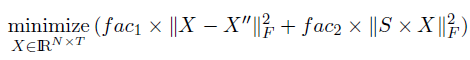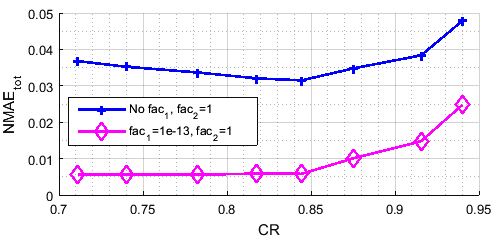# A minimization of a function with two weighted terms using the semidefinte programming and CVX package

Hi everybody,

I have to minimize w1ObjA(X) + w2ObjB(X) to get X. I used the SDP with the CVX package for the resolution. When w1 is chosen be very small (10^-13) and w2=1. The minimization gave us good results.
The same things (same results) are happened when w1=0, which is unexpectable.

However, when we have completely removed the first term and we have minimized only w2*ObjB(X), we did not have the same results. Instead, we obtained bad performance, which is expectable for our case.

When we put w=0, did the SDP with the CVX package continue considering the first term when resolving w1ObjA(X) + w2ObjB(X) ?? I think that it all depends on how the procedure works internally.

I doubt anyone based on the information you provide can say something clever. I mean what is bad performance for instance.

You could for instance post log output for the different runs.

Btw why do you need a small weight of 1.0e-13. That almost screams about that you have numerical issues in your model.

You are right, I think I did not explain the problem well.
The minimization that I use is for recovering the data X from another data matrix that holds some missing entries.
I mean by “we obtained bad performance” that we obtained a high data recovery error.

Most likely your model or data is “broken”. You are unlikely to get a useful answer unless you provide more info e.g. the optimizer logs.

Ok, the function that we would minimize is as follows:X_second and S are two known matrices. X_second is the data matrix, which holds some missing entries. S holds the similarities weights between data rows. fac1 and fac2 are respectively w1 and w2.
The matlab code that I use is as follows:
cvx_begin
variable X(N,T)
minimize( fac_frst*(sum(sum_square(X-X_second)))+ fac_secd* (sum(sum_square(S*X))) );
cvx_end

Here, it is expectable that when we completely remove the first term, the data recovery error for X gets worse since X_second serves as a database to fill the missing data as it already holds data values in the different order of magnitudes that are available on the data field.

However, when we put fac1=0, we do not obtain the same result (data recovery error) as when we completely remove the first term.
My question is : Did the SDP with the CVX package continue considering the first term even when we put fac1=0 ?
Someone told me that it may depend on how the procedure works internally. For that reason, I resort to this forum.
I hope that you can help me to understand this issue since I’m not expert in mathematics.

I presume `fac1` means `fac_first`?

If so, the whether the term is removed or fac = 0 should not make a difference in the optimal objective value (within tolerance), but perhaps (I don;t know) the solver is not sent the exact same problem by CVX, and so might pick a different solution having the same objective value (within tolerance). If you have numerically bad data, perhaps this won’t be true.

You haven’t even shown the complete programs, with complete CVX and solver output, and illustration of differences in solution. So we are having to speculate, and don’t really know what is going on.

1 Like

Your request is quite typical for questions. So please allow me to analyze it a bit.

Let us try to think what the possible problem could be. Here are the 3 posibilities:

1. A bug in the software.
2. The optimizer you using does not compute an accurate solution.
3. Your model/data is somewhat broken.

Regarding 1 then it is unlikely and in any case you provide zero evidence that should be case. Or any possibility for us diagnose it. We do not even know what optimizer you using e.g. sedumi.

Regarding 2 then it might be possible but we cannot say anything about this since we do not have optimizer logs or the problem so we can analyze it. In any case we do not know the optimizer you are using.

Regarding 3 then we have some idea about what your problem is but no data to reproduce the issue. We know you get vastly different results for fac1 0.0 and 1.0e-13. A problem is illposed if the solution change a lot for small perturbation in the input data. So given the very limited information concluding your problem is illposed seems the best we can do. Why

Although people like @Mark_L_Stone has big modelling zen then it is close to impossible for us to provide useful help.

PS.

Why do not you fix fac2 to 1 and then adjust fac1 accordingly. Also why do you square the norms. In expirience the conditioning worse.Maybe you can choose a more sensible fac1 if you do not square.

1 Like

For the optimizer, I have downloaded the “Standard bundles, including Gurobi and/or MOSEK”, the one for Windows. Normally, I don’t have a bug in the software since it works very well.

As for 3, contrariwise, when fac1=0 or fac1=1e-13, we obtain exactly the same data recovery error for X. The problem is when we completely remove the first term and minimize only the term that is related to fac2, we do not obtain the same result (data recovery error) as when we put fac1=0.

Regarding your remark, that’s what I have exactly done, I have fixed fac2 to 1, then I have varied fac1 until I got the best data recovery error with fac1=1e-13 and even I decrease more fac1, I still obtain the same result.

Yes fac1 is fac_first. The complete program is very long with multiple of functions. But, I assure you that the code used to generate the data to be manipulated is well validated.

The results are as follows:
The first figure depicts the results when we have removed the first term that is related to fac1.
The seconf figure depicts the results when we have put fac1=0.Please post full log outputs from the solves. It has been said a few times, but I will try again. Without it all answers are completely speculative. If you post the logs, the answers will still be guesses, but a bit more educated. So please post full log outputs. Especially as we still don’t know for sure which solver you are using.

It is understandable that you focus on the final answers you get but to diagnose it it is also necessary to know about the whole solution process. So please post log outputs.

Regarding your other question, having fac1=0 and not having that term at all can of course generate different optimizer models, because cvx may still generate code needed to represent that term, even if it then appears in the objective with zero coefficient. In the perfect mathematical world this should not make a difference, but… Again, this is speculation. If you want to inspect what model is generated, there are cvx options to dump the low-level model to a file. Then it will be very easy to deduce what happens.

1 Like

PS. Here is a small experiment for you. I ran

``````cvx_begin
variable x(1)
minimize 0*norm(x-1)
subject to
x <= 3
cvx_solver mosek
cvx_solver_settings('write', 'dump.opf')
cvx_end
``````

And you can see that the conic representation of norm is generated in the model, it just enters the objective with coefficient 0. So that model is only theoretically equivalent to a model with empty objective.

1 Like

Dear Michal,
It turned out that I did not understand their request. I hope that I have provided now what has been requested and I apologize for this misunderstanding.

For the same data compression ratio, the log outputs are as follows:

When we put fac1=0:

when we remove the first term that is related to fac1:

I don’t know what you were plotting, but …

The optimal objective values are all basically (within solver tolerance) zero, so you’re just getting (apparently) different values of X which achieve that. I say “apparently” because you haven’t shown us what those differences are.

I don’t know what the relation is between the data recovery error, which is what you seem to really care about, and the objective function provided to CVX. If equal objective values in CVX can be associated with very different data recovery errors, then perhaps your optimization problem modeling is not up to snuff for your intended use.

You are using SDP3 to solve the problem. To me it seems SDPT3 solved your problem nicely. No numerical problem.

The only strange thing is that SDPT3 says the objective value is -100 internally but the reported objective value of almost 0.

You could try Mosek just to see what happens.

Because of transformations applied by CVX,tThe objective value of the problem provided by CVX to the solver (which is displayed in the solver log) is not necessarily the same as the objective value of the problem entered by the user (which is what is shown as cvx_optval).

I’m plotting the data recovery error, which is the Normalized Mean Absolute Error on all missing entries (NMAE_tot) between the recovred data matrix X (obtained through the aforementioned minimization) and the original data matrix (the raw data matrix). Namely, I find X through the mnimization , then I compute the data recovery error, and the lower NMAE_tot that we obtain, the more efficient the minimization process is. (We aim to reach a close fit to the matrix X_second, which holds the elements of the original data matrix with may missing data entries that we want to recover).

To search for the appropriate tuning parameters, I have fixed fac2 to 1, then I have varied fac1 until I got the best data recovery error (the lowest one) with fac1=1e-13.

SDPT3 probably look for 6 to 8 figures accuracy in the optimal objective value.

Since SDPT3 sees the optimal objective value as -100.0 then that might translate into a relative large error in the final CVX objective if it is close to 0 in absolute value.

It might be fac1=1.0e-13 gives the best error, but using such a small penalty is unlikely to be robust.